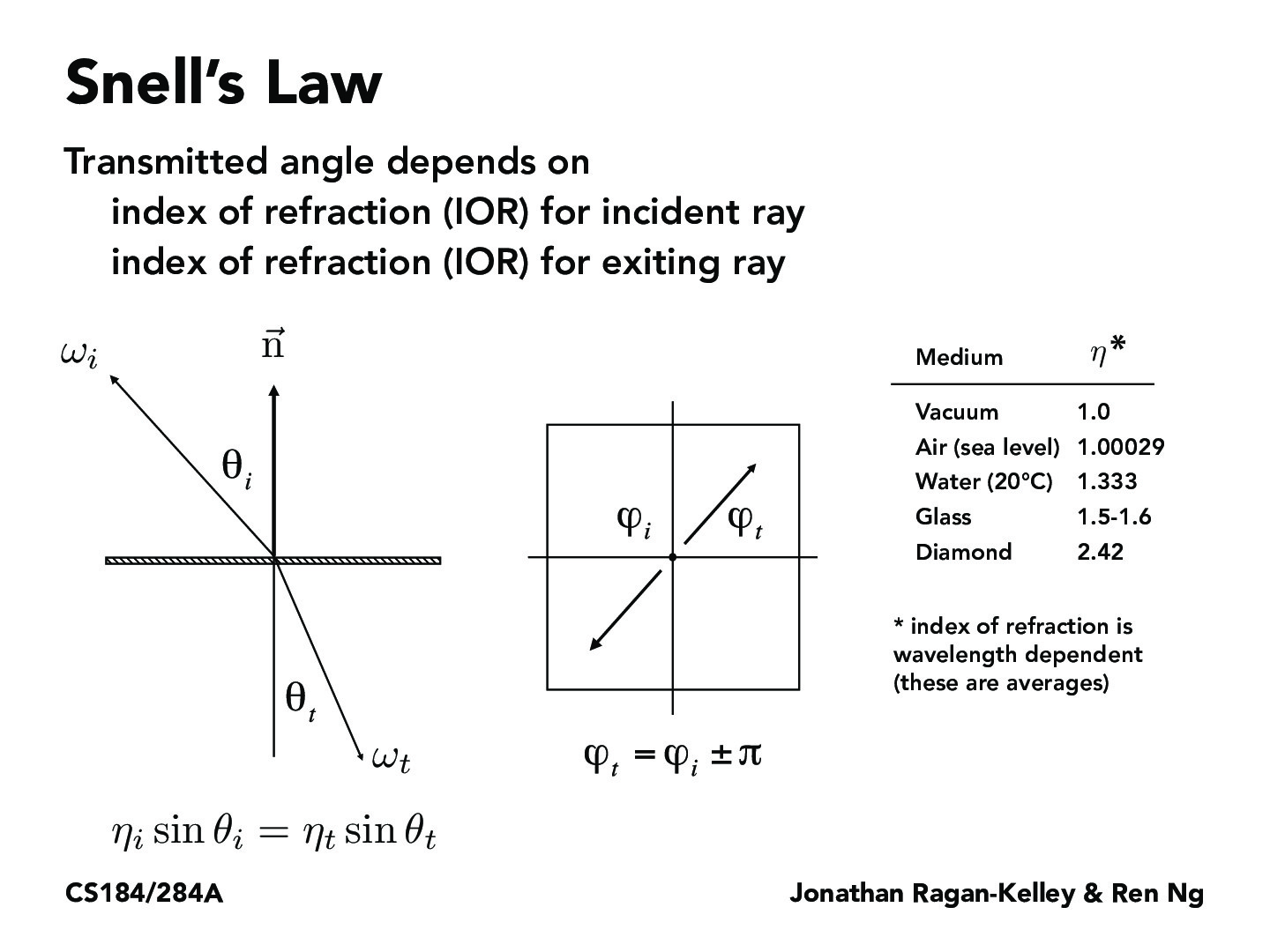You are viewing the course site for a past offering of this course. The current offering may be found here.Lecture 14: Material Modeling (20)rutajoshi

What are phi_i and phi_t in this case? If they are the indices of refraction, what is the 2x2 graph on the right supposed to represent?sirejdua

Similar to slide 16, this box represents the top down view of these rays. The condition that phi_t = phi_i +- pi means that from the top down view, these two projected vectors appear to be antiparallel.x-fa19

The equation eta_i * sin(theta_i) = eta_t * sin(theta_t) represents the relation that the incident angle with respect to the normal has with the transmitted angle with respect to the normal in the opposite direction. Eta represents the indices of refraction of the material in question; materials such as diamonds that glitter dramatically have a high index of refraction.Jordanwyli

I'm kinda confused why the two vectors, wi and wt, both point outwards from the intersection point. This convention seems confusing to me. Could someone explain why this is preferred? Is it so that we can easier find the angle between each vector and the normal?orkun1675

Intuition: as the material gets denser the light ray will get closer to the normal because if eta increases theta should decrease to keep the ratio. So light going into a diamond from the air will be bent closer to the surface normal.irisli

There is this weird symbol:

$\eta$

It's called eta but it's H. This is the same H in HKN. So weird.

Wikipedia and many places just use the letter "n" instead of $\eta$.

You must be enrolled in the course to comment